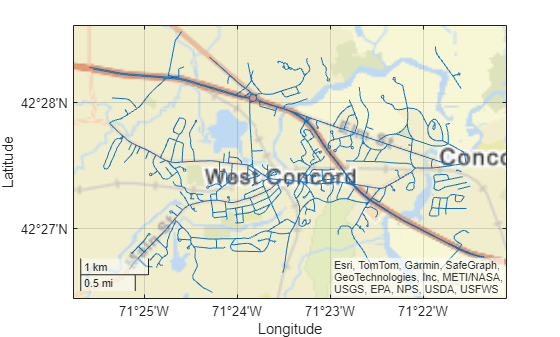# maplineshape

Line shape in planar coordinates

## Description

A `maplineshape` object represents a line or multiline in planar coordinates. A multiline is an individual line shape that contains a set of separate lines.

To represent a line or multiline in geographic coordinates, use a `geolineshape` object instead.

## Creation

To create `maplineshape` objects, either:

• Import line data in planar coordinates as a geospatial table using the `readgeotable` function, and then query the `Shape` variable of the table.

• Use the `maplineshape` function (described here).

### Syntax

``shape = maplineshape(x,y)``

### Description

example

````shape = maplineshape(x,y)` creates a `maplineshape` object or array of `maplineshape` objects with vertices at the specified x- and y-coordinates. The sizes of `x`, `y`, and the `maplineshape` object array `shape` match.```

### Input Arguments

expand all

x-coordinates, specified as a numeric vector or a cell array of numeric vectors.

• Create a line by specifying a vector, such as ```[65 62 53 66]```.

• Create a multiline by specifying a vector and including line breaks as `NaN` values, such as ```[55 34 18 NaN 14 19 42 26]```.

• Create an array of lines and multilines by specifying a cell array of vectors, such as `{[55 34 18],[14 19 NaN 42 26 31]}`.

The `NaN` values in `x` must correspond to the `NaN` values in `y`.

The size of `x` must match the size of `y`. For cell arrays, the size of the vector in each cell of `x` must match the size of the vector in the corresponding cell of `y`.

Data Types: `double` | `cell`

y-coordinates, specified as a numeric vector or a cell array of numeric vectors.

• Create a line by specifying a vector, such as ```[4 59 121 98]```.

• Create a multiline by specifying a vector and including line breaks as `NaN` values, such as ```[78 56 63 NaN 83 106 104 126]```.

• Create an array of lines and multilines by specifying a cell array of vectors, such as ```{[78 56 63],[83 106 NaN 104 126 131]}```.

The `NaN` values in `x` must correspond to the `NaN` values in `y`.

The size of `x` must match the size of `y`. For cell arrays, the size of the vector in each cell of `x` must match the size of the vector in the corresponding cell of `y`.

## Properties

expand all

Number of line parts, returned as an array of nonnegative integers.

For a `maplineshape` scalar, the value of `NumParts` is `1` when the `maplineshape` object represents a single line and more than `1` when the object represents a multiline.

For a `maplineshape` array, the size of `NumParts` matches the size of the array.

Data Types: `double`

Geometric type, returned as `"line"`.

Data Types: `string`

Coordinate system type, returned as `"planar"`.

Data Types: `string`

Projected coordinate reference system (CRS), specified as a `projcrs` object. A projected CRS consists of a geographic CRS and several parameters that are used to transform coordinates to and from the geographic CRS.

## Object Functions

 `geoplot` Plot points, lines, and polygons in geographic axes `mapclip` Clip shape to xy-limits in planar coordinates

## Examples

collapse all

Import a shapefile containing a network of road segments in Concord, MA as a geospatial table. The shapefile represents the road segments using lines. Get information about the fourth line by querying the `Shape` variable of the table.

```GT = readgeotable("concord_roads.shp"); GT.Shape(4)```
```ans = maplineshape with properties: NumParts: 1 Geometry: "line" CoordinateSystemType: "planar" ProjectedCRS: [1×1 projcrs] ```

Display the road segments on a street map.

```geoplot(GT) geobasemap streets```Create an individual line as a `maplineshape` scalar. Specify the projected CRS as World Equidistant Cylindrical, which has the EPSG authority code `4087`.

```x = [4 59 121 98]; y = [65 62 53 66]; lineshp = maplineshape(x,y); p = projcrs(4087); lineshp.ProjectedCRS = p```
```lineshp = maplineshape with properties: NumParts: 1 Geometry: "line" CoordinateSystemType: "planar" ProjectedCRS: [1x1 projcrs] ```

Create a multiline as a `maplineshape` scalar.

```x = [78 56 63 NaN 83 106 104 126]; y = [55 34 18 NaN 14 19 42 26]; multiline = maplineshape(x,y); multiline.ProjectedCRS = p```
```multiline = maplineshape with properties: NumParts: 2 Geometry: "line" CoordinateSystemType: "planar" ProjectedCRS: [1x1 projcrs] ```

Create one individual line and one multiline as a 1-by-2 `maplineshape` array.

```x = {[78 56 63],[83 106 NaN 104 126 113]}; y = {[55 34 18],[14 19 NaN 42 26 37]}; lineMultiline = maplineshape(x,y); lineMultiline.ProjectedCRS = p```
```lineMultiline=1×2 object 1x2 maplineshape array with properties: NumParts: [1 2] Geometry: "line" CoordinateSystemType: "planar" ProjectedCRS: [1x1 projcrs] ```

## Version History

Introduced in R2021b